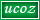Monday, 2019-05-20, 2:39 PM CBSE GUIDE
 Welcome Guest | RSS
 MATHEMATICS       CBSE SAMPLE PAPERS FOR MATHEMATICS CLASS 10TH   CBSE sample papers for class 10th students for MATHEMATICS. We are providing a wide range of sample papers for the CBSE students for MATHEMATICS for the better practice of what they have learnt.   MATHEMATICS SAMPLE PAPER 1               Based on CBSE pattern.             Free sample papers for CBSE students on MATHEMATICS.       MATHEMATICS SAMPLE PAPER 2               Based on CBSE pattern.             Free sample papers for CBSE students on MATHEMATICS.       MATHEMATICS SAMPLE PAPER 3               Based on CBSE pattern.             Free sample papers for CBSE students on MATHEMATICS.       MATHEMATICS SAMPLE PAPER 4               Based on CBSE pattern.             Free sample papers for CBSE students on MATHEMATICS.       MATHEMATICS SAMPLE PAPER 5               Based on CBSE pattern.             Free sample papers for CBSE students on MATHEMATICS.       MATHEMATICS SAMPLE PAPER 6               Based on CBSE pattern.             Free sample papers for CBSE students on MATHEMATICS.       MATHEMATICS SAMPLE PAPER 7               Based on CBSE pattern.             Free sample papers for CBSE students on MATHEMATICS.       MATHEMATICS SAMPLE PAPER 8               Based on CBSE pattern.             Free sample papers for CBSE students on MATHEMATICS.       MATHEMATICS SAMPLE PAPER 9               Based on CBSE pattern.             Free sample papers for CBSE students on MATHEMATICS.       MATHEMATICS SAMPLE PAPER 10               Based on CBSE pattern.             Free sample papers for CBSE students on MATHEMATICS.            DOWNLOAD    MATHEMATICS SAMPLE PAPER 11               Based on CBSE pattern.             Free sample papers for CBSE students on MATHEMATICS.
 StatisticsTotal online: 1 Guests: 1 Users: 0Copyright CBSEguide © 2019